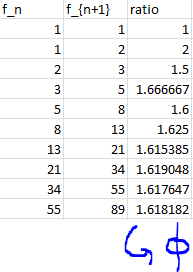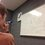# The Golden Ratio: Fibonacci RatiosHere is the previous post concerning the Golden Ratio. For a collection of all the posts concerning the Golden Ratio, click #GoldenRatio below.

Now we can talk about the Fibonacci Numbers and the Golden Ratio. As Sharky presented in the previous post, we can take the successive ratios of Fibonacci numbers and get something interesting. See the picture above. The ratios approach the golden ratio! Now that we have found an interesting fact, let's try and prove it. How do we know that they converge to the golden ratio and not some other number?

First, let's assume that the ratios converge to some number $x$. Then, for really large $n$, $\frac{f_{n+1}}{f_n} = x$. Using the definition of the Fibonacci sequence we have

$x = \frac{f_{n+1}}{f_n} = \frac{f_{n-1}+f_n}{f_n} = \frac{f_{n-1}}{f_n} + 1 = \frac{1}{x} +1$

This should be a very familiar equation! From this we know that $x=\phi$. But there are still some problems in this proof. It is more rigorous than our original derivation, but it still is based off of many assumptions. We already assume that the ratios converge. We must prove this first to be truly rigorous. Also, not even for very large $n$ is $\frac{f_{n+1}}{f_n} = x$ true. The ratios never actually reach $\phi$, but only approach it. Thus assumption simplified the proof, but also made it less rigorous. All in all, a better approach would be more helpful. But we will have to wait for this until we know a bit more about the nature of the Fibonacci sequence.

Take a look again at our proof though. Did it depend on which values we started? No. Thus we can conclude that any general Fibonacci-like sequence will have ratios that converge to the golden ratio.

On another note, remember the continued fraction representation of the golden ratio? Well, I want you to try an experiment. Get a calculator, and type in any nonzero number. Take the reciprocal of that number, and add 1. Take the reciprocal of that number again, and add 1. Continue this until you notice something interesting...

These set of operations take any nonzero number and converge towards the golden ratio. This is seen in the continued fraction representation, for in each step of the continued fraction, we are taking the reciprocal of what we already had, and adding 1. Except, with the method I described, its like we are starting our way at the infinity end and working our way back up. Any way, this pattern can be reflected in $\phi = \frac{1}{\phi} +1$. And even more interestingly, it can be seen in the proof above.

Try this same sort of idea with the infinite radical representation of $\phi$. The same sort of thing should happen.

Is there any other interesting things that you can derive?

Here is the next post.Note by Bob Krueger
7 years, 6 months ago

This discussion board is a place to discuss our Daily Challenges and the math and science related to those challenges. Explanations are more than just a solution — they should explain the steps and thinking strategies that you used to obtain the solution. Comments should further the discussion of math and science.

When posting on Brilliant:

• Use the emojis to react to an explanation, whether you're congratulating a job well done , or just really confused .
• Ask specific questions about the challenge or the steps in somebody's explanation. Well-posed questions can add a lot to the discussion, but posting "I don't understand!" doesn't help anyone.
• Try to contribute something new to the discussion, whether it is an extension, generalization or other idea related to the challenge.

MarkdownAppears as
*italics* or _italics_ italics
**bold** or __bold__ bold
- bulleted- list
• bulleted
• list
1. numbered2. list
1. numbered
2. list
Note: you must add a full line of space before and after lists for them to show up correctly
paragraph 1paragraph 2

paragraph 1

paragraph 2

[example link](https://brilliant.org)example link
> This is a quote
This is a quote
    # I indented these lines
# 4 spaces, and now they show
# up as a code block.

print "hello world"
# I indented these lines
# 4 spaces, and now they show
# up as a code block.

print "hello world"
MathAppears as
Remember to wrap math in $$ ... $$ or $ ... $ to ensure proper formatting.
2 \times 3 $2 \times 3$
2^{34} $2^{34}$
a_{i-1} $a_{i-1}$
\frac{2}{3} $\frac{2}{3}$
\sqrt{2} $\sqrt{2}$
\sum_{i=1}^3 $\sum_{i=1}^3$
\sin \theta $\sin \theta$
\boxed{123} $\boxed{123}$

Sort by:

Nice article.

Here are some questions to consider (esp for Level 3's reading this)

1) "First, let's assume that the ratios converge to some number $x$ ... This assumption simplified the proof, but also made it less rigorous".

Can you prove the assumption? Must it always be true?

2) We know that this ratio must satisfy the equation $x = 1 + \frac{1}{x}$. Does this necessarily imply that it must be equal to $\phi$? Why, or why not?

3) Does this proof work if we allow for negative starting values? In particular, is it possible for $f_n$ to be 0 infinitely often?

Staff - 7 years, 6 months ago

Thanks! I tried to come up with a not-intentionally-fallacious "proof" that would purposely serve as a bad example.

- 7 years, 6 months ago